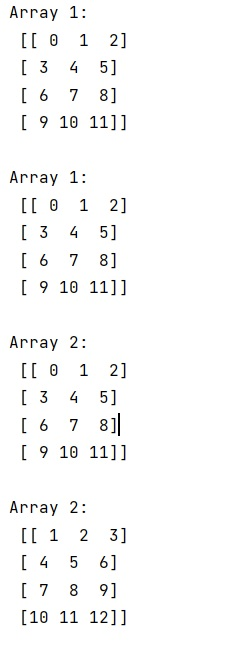# What is the difference between i+1 and i += 1 in a for loop with NumPy?

Learn the difference between i+1 and i += 1 in a for loop with NumPy in Python?
Submitted by Pranit Sharma, on January 05, 2023

NumPy is an abbreviated form of Numerical Python. It is used for different types of scientific operations in python. Numpy is a vast library in python which is used for almost every kind of scientific or mathematical operation. It is itself an array which is a collection of various methods and functions for processing the arrays.

## Difference between i+1 and i += 1 in a for loop

In an incremental for loop i = i + 1 reassigns i, i += 1 increments i by 1. The difference is that one modifies the data-structure itself (in-place operation) b += 1 while the other just reassigns the variable a = a + 1.

But, i += 1 is not always doing an in-place operation, there are (at least) three exceptions:

• If xdoes not implement an __iadd__ method then the i += 1 statement is just a shorthand for i = i + 1. This would be the case if i was something like an int.
• If __iadd__returns NotImplemented, Python falls back to i = i + 1.
• The __iadd__method could theoretically be implemented to not work in place. It would be really weird to do that, though.

As it happens your bs are numpy.ndarrays which implements __iadd__ and return itself so your second loop modifies the original array in-place.

Let us understand with the help of an example,

## Python code to demonstrate the difference between i+1 and i += 1 in a for loop

```# Import numpy
import numpy as np

# Creating an array
arr = np.arange(12).reshape(4,3)

# Display original array
print("Array 1:\n",arr,"\n")

# Using for loop with a = a+1
for i in arr:
i = i + 1

# Display arr
print("Array 1:\n",arr,"\n")

# Creating an array
arr2 = np.arange(12).reshape(4,3)

# Display arr 2
print("Array 2:\n",arr,"\n")

# Using for loop with a+=1
for i in arr2:
i+=1

# Display arr 2
print("Array 2:\n",arr2,"\n")
```

Output: